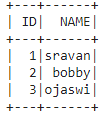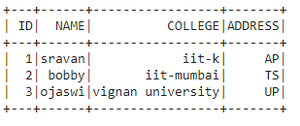Related Articles

# How to create a PySpark dataframe from multiple lists ?

• Last Updated : 30 May, 2021

In this article, we will discuss how to create Pyspark dataframe from multiple lists.

### Approach

• Create data from multiple lists and give column names in another list. So, to do our task we will use the zip method.

zip(list1,list2,., list n)

• Pass this zipped data to spark.createDataFrame() method

dataframe = spark.createDataFrame(data, columns)

### Examples

Example 1: Python program to create two lists and create the dataframe using these two lists

## Python3

 `# importing module``import` `pyspark`` ` `# importing sparksession from ``# pyspark.sql module``from` `pyspark.sql ``import` `SparkSession`` ` `# creating sparksession and giving ``# an app name``spark ``=` `SparkSession.builder.appName(``'sparkdf'``).getOrCreate()`` ` `# list  of college data with dictionary``# with two lists in three elements each``data ``=` `[``1``, ``2``, ``3``]``data1 ``=` `[``"sravan"``, ``"bobby"``, ``"ojaswi"``]`` ` `# specify column names``columns ``=` `[``'ID'``, ``'NAME'``]`` ` `# creating a dataframe by zipping the two lists``dataframe ``=` `spark.createDataFrame(``zip``(data, data1), columns)`` ` `# show data frame``dataframe.show()`

Output:Example 2: Python program to create 4 lists and create the dataframe

## Python3

 `# importing module``import` `pyspark`` ` `# importing sparksession from ``# pyspark.sql module``from` `pyspark.sql ``import` `SparkSession`` ` `# creating sparksession and giving ``# an app name``spark ``=` `SparkSession.builder.appName(``'sparkdf'``).getOrCreate()`` ` `# list  of college data with dictionary``# with four lists in three elements each``data ``=` `[``1``, ``2``, ``3``]``data1 ``=` `[``"sravan"``, ``"bobby"``, ``"ojaswi"``]``data2 ``=` `[``"iit-k"``, ``"iit-mumbai"``, ``"vignan university"``]``data3 ``=` `[``"AP"``, ``"TS"``, ``"UP"``]`` ` `# specify column names``columns ``=` `[``'ID'``, ``'NAME'``, ``'COLLEGE'``, ``'ADDRESS'``]`` ` `# creating a dataframe by zipping ``# the two lists``dataframe ``=` `spark.createDataFrame(``  ``zip``(data, data1, data2, data3), columns)`` ` `# show data frame``dataframe.show()`

Output:Attention geek! Strengthen your foundations with the Python Programming Foundation Course and learn the basics.

To begin with, your interview preparations Enhance your Data Structures concepts with the Python DS Course. And to begin with your Machine Learning Journey, join the Machine Learning – Basic Level Course

My Personal Notes arrow_drop_up